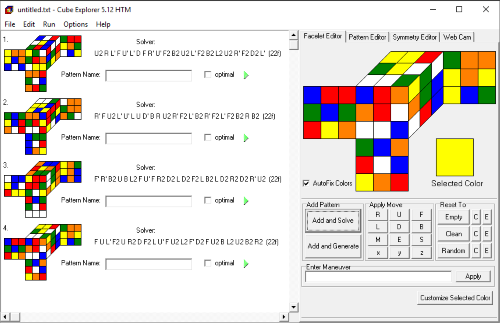# Herbert Kociemba’s Optimal Cube Solver – Cube Explorer

Ever since the Rubik's Cube’s debut many decades ago, it has posed many questions far beyond the solving of it. One of these puzzles has been the optimal solution for a given scrambled state. Once we had solved the puzzle, we wanted to push it further. How can we solve any given scrambled cube in the least number of moves possible? Mathematics and several decades of research has proved that any scramble can be solved in under 20 moves. But how do we find the smallest combination of moves? The answer resides in Herbert Kociemba's Cube Explorer program.The desktop version of Cube Explorer

Let’s first take a look at the long winded approach – a cube solver that would take a scrambled cube and find the shortest sequence of moves required to solve it. Since the value must be <20 and there is no collection of the exact number of moves for each of the 43 quintillion possibilities, let’s use results from the Cube Explorer itself – Out of 100,000 unique scrambles, 93,772 of these had minimums of 17 or 18 moves (26,673 and 67,099 respectively). Therefore we shall use an approximation of 17.75 moves per solve. There are 18 different possible moves, and if we assume that, following the completion of a move, the following move must be performed on a different layer (reducing the possible move number to 15), then there are 18 x 15^16.75 possible combinations of moves, of which the shortest one would have to be found. For every single scramble, approximately 901,158,314,000,000,100,000 (901 quintillion) solutions would have to be checked. This would take an immense amount of hours for even some of the best machinery; keeping in mind that even the 43 quintillion possible scrambles is too big a number to process, hence the Cube Explorer’s test with 100,000 scrambles.

So how exactly do we find the shortest number of moves to solve a scramble without checking an immensely large number of possible moves? It all starts with the Two-Phase-Algorithm.

## The Two-Phase-Algorithm

Phase 1 of the Two-Phase-Algorithm uses a set of the 18 possible moves that, regardless of order or number of moves, cannot change the orientation of edge and corner pieces – G1. This set includes <U D R2 F2 L2 B2>, and it also means that pieces within the U and D layers cannot move out of the two layers (only 2 moves can be performed which would simply move pieces from U to D and vice-versa). Since this is a subset of all possible scrambles (a subset which has a large number of acceptable outputs), it is much easier to get to than going from a scrambled cube to a solved one in one step. An algorithm is performed which increases the count of allowed moves used to find a solution that takes the cube to a G1 state. Once it finds the shortest number of moves required to do this, it continues until it has a large enough supply of possible solutions ranging from the lowest number of moves to a much larger number.

In Phase 2, the permutations of the edges and corners are restored. However, G1 is still an incredibly large subset; it just wouldn’t be possible to do all of this accurately. Therefore, an estimate is made on the number of moves required for Phase 2. The algorithm continues to find shorter and shorter solutions by using some not-so-optimal Phase 1 values that produce more optimal Phase 2 values. For example, an 8 move Phase 1 followed by a 15 move Phase 2 is less optimal than a 10 move Phase 1 followed by a 5 move Phase 2. As the Phase 1 value increases, the Phase 2 value decreases. Eventually, a solution will be found where the value of Phase 2 is 0 – This is the optimal solution.

Of course, there could be other solutions where Phase 2 is needed, however the implementation above is much more efficient and provides very accurate results.

Symmetry is also another concept used in the Cube Explorer. A cube can be rotated and produce a different case. Therefore geometrical transformation can be applied to cubes to drastically reduce the number of possible cases and enhancing the solver.

For picture cubes, the centre piece’s orientation is vital otherwise the cube is not “solved”. There are 2048 different centre combinations, and Kociemba proved that any picture cube could be solved in 21 moves or less.